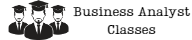# Using Monte Carlo Simulation to Evaluate Risk and Uncertainty in Data

In today’s data-driven world, businesses and organizations rely heavily on data to make informed decisions. However, data is never perfect and often comes with degrees of uncertainty and risk. In order to make accurate decisions, it is crucial to evaluate these uncertainties and risks. One way to do this is through Monte Carlo simulation. In this article, we will explore what Monte Carlo simulation is, how it works, and how it can be used to evaluate risk and uncertainty in data.## What is Monte Carlo Simulation?

Monte Carlo simulation is a computational technique that uses random sampling to simulate real-world scenarios. It is named after the famous Monte Carlo Casino in Monaco, where games of chance rely heavily on randomness. The technique was first introduced in the 1940s by scientists working on the Manhattan Project, who used it to simulate the behavior of neutrons in a nuclear reactor.

In Monte Carlo simulation, a model is created that describes the behavior of a system. The model includes all the variables that affect the system and their relationships. Random values are then assigned to these variables, and the model is run multiple times to generate a distribution of possible outcomes. The distribution represents the range of possible outcomes and their probabilities.

## How Does Monte Carlo Simulation Work?

Monte Carlo simulation works by generating random samples from a probability distribution. The probability distribution reflects the uncertainty and variability of the variables in the model. The samples are then used to calculate the outcome of the model. This process is repeated many times to generate a distribution of possible outcomes.

For example, let’s say we want to simulate the performance of a stock portfolio. We would create a model that includes variables such as the expected return of each stock, the standard deviation of the returns, and the correlation between the stocks. We would then assign random values to these variables and run the model. We would repeat this process many times to generate a distribution of possible portfolio returns.

## Benefits of Monte Carlo Simulation

Monte Carlo simulation has several benefits. One of the main benefits is that it allows us to evaluate the risk and uncertainty of a system. By generating a distribution of possible outcomes, we can see the range of possible results and their probabilities. This information can be used to make informed decisions and manage risk.

Another benefit of Monte Carlo simulation is that it can handle complex systems with many variables. Traditional analytical methods may not be able to handle the complexity of these systems, but Monte Carlo simulation can. This makes it a powerful tool for decision-making in fields such as finance, engineering, and science.

## Using Monte Carlo Simulation in Finance

Monte Carlo simulation is widely used in finance for risk management, portfolio optimization, and option pricing. In risk management, it can be used to evaluate the risk of a portfolio of investments. By simulating the possible outcomes of the portfolio, we can see the range of possible losses and their probabilities. This information can be used to make informed decisions about how to manage the risk of the portfolio.

In portfolio optimization, Monte Carlo simulation can be used to find the optimal mix of investments that maximizes return while minimizing risk. By simulating the possible outcomes of different portfolios, we can find the one that provides the best risk-return tradeoff.

In option pricing, Monte Carlo simulation can be used to price options that are too complex for traditional analytical methods. By simulating the possible outcomes of the underlying asset, we can calculate the value of the option.

## Conclusion

Monte Carlo simulation is a powerful technique for evaluating risk and uncertainty in data. It allows us to generate a distribution of possible outcomes and their probabilities, which can be used to make informed decisions. Monte Carlo simulation is widely used in finance, engineering, and science to manage risk, optimize portfolios, and price complex options. By using Monte Carlo simulation, businesses and organizations can make better decisions and manage risk more effectively.

### Try some of our classes

Enter your email and we’ll send you some samples of our favorite classes.

#### Course Categories

Quisque velit nisi, pretium ut lacinia in, elementum id enim.